• +91 9971497814
• info@interviewmaterial.com

# Chapter 2 Relations and Functions Ex-2.3 Interview Questions Answers

### Related Subjects

Question 1 :
Which of the following relations are functions? Give reasons. If it is a function, determine its domain and range.
(i) {(2, 1), (5, 1), (8, 1), (11, 1), (14, 1), (17, 1)}
(ii) {(2, 1), (4, 2), (6, 3), (8, 4), (10, 5), (12, 6), (14, 7)}
(iii) {(1, 3), (1, 5), (2, 5)}

(i) {(2, 1), (5, 1), (8, 1), (11, 1), (14, 1), (17, 1)}
As 2, 5, 8, 11, 14, and 17 are the elements of the domain of the given relation having their unique images, this relation can be called as a function.
Here, domain = {2, 5, 8, 11, 14, 17} and range = {1}
(ii) {(2, 1), (4, 2), (6, 3), (8, 4), (10, 5), (12, 6), (14, 7)}
As 2, 4, 6, 8, 10, 12, and 14 are the elements of the domain of the given relation having their unique images, this relation can be called as a function.
Here, domain = {2, 4, 6, 8, 10, 12, 14} and range = {1, 2, 3, 4, 5, 6, 7}
(iii) {(1, 3), (1, 5), (2, 5)}
It’s seen that the same first element i.e., 1 corresponds to two different images i.e., 3 and 5, this relation cannot be called as a function.

Question 2 :
Find the domain and range of the following real function:
(i) f(x) = –|x| (ii) f(x) = √(9 – x2

(i) Given,

f(x) = –|x|, x  R

We know that,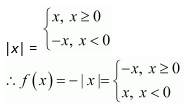As f(x)is defined for x  R, the domain of f is R.

It is also seen thatthe range of f(x) = –|x| is all real numbers exceptpositive real numbers.

Therefore, the rangeof f is given by (–∞, 0].

(ii) f(x) = √(9 –x2)

As √(9 – x2) isdefined for all real numbers that are greater than or equal to –3 and less thanor equal to 3, for 9 – x2 ≥ 0.

So, the domainof f(x) is {x: –3 ≤ x ≤ 3} or [–3,3].

Now,

For any value of x inthe range [–3, 3], the value of f(x) will lie between 0 and3.

Therefore, the rangeof f(x) is {x: 0 ≤ x ≤ 3} or [0, 3].

Question 3 :
A function f is defined by f(x) = 2x – 5. Write down the values of
(i) f(0), (ii) f(7), (iii) f(–3)

Given,
Function, f(x) = 2x – 5.
Therefore,
(i) f(0) = 2 × 0 – 5 = 0 – 5 = –5
(ii) f(7) = 2 × 7 – 5 = 14 – 5 = 9
(iii) f(–3) = 2 × (–3) – 5 = – 6 – 5 = –11

Question 4 :
The function ‘t’ which maps temperature in degree Celsius into temperature in degree Fahrenheit is defined by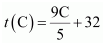.
Find (i) t (0) (ii) t (28) (iii) t (–10) (iv) The value of C, when t(C) = 212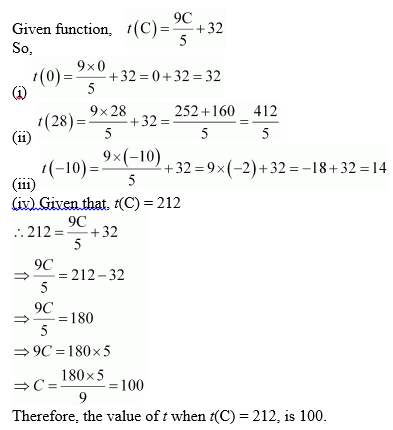Question 5 :
Find the range of each of the following functions.
(i) f(x) = 2 – 3x, x ∈ R, x > 0.
(ii) f(x) = x2 + 2, x is a real number.
(iii) f(x) = x, x is a real number.

(i) Given,
f(x) = 2 – 3x, x ∈ R, x > 0.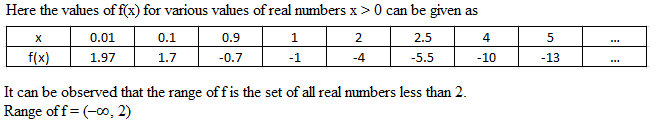We have,
x > 0
So,
3x > 0
-3x < 0 [Multiplying by -1 both the sides, the inequality sign changes]
2 – 3x < 2
Therefore, the value of 2 – 3x is less than 2.
Hence, Range = (–∞, 2)
(ii) Given,
f(x) = x2 + 2, x is a real number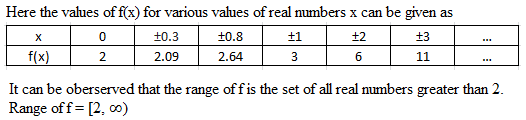We know that,

x2 ≥ 0

So,

x2 + 2 ≥ 2 [Adding 2 both the sides]

Therefore, the valueof x2 + 2 is always greater or equal to 2 for x isa real number.

Hence, Range = [2, ∞)

(iii) Given,

f(x) = x, x is a realnumber

Clearly,the range of f is the set of all real numbers.

Thus,

Range of f = R

Todays Deals### Chapter 2 Relations and Functions Ex-2.3 Contributorskrishan

Name:
Email:

# Latest News# 9000 interview questions in different categories